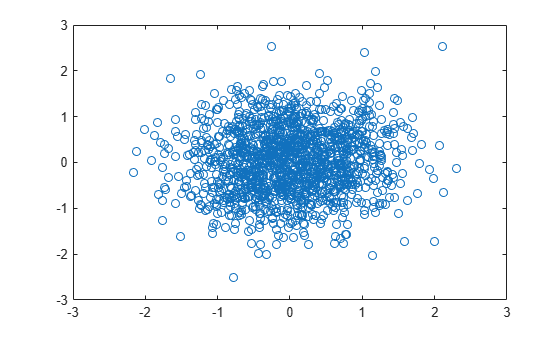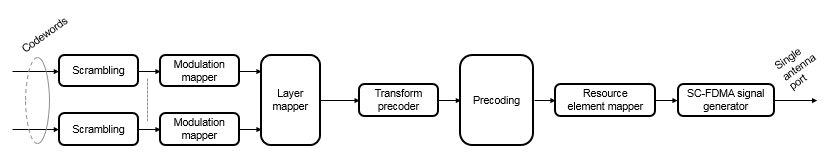ltePSSCH

Description

example

sym = ltePSSCH(ue,cw) returns a complex symbol column vector containing the physical sidelink shared channel (PSSCH) for the specified UE settings structure and codeword bits. Channel processing performed by the function includes PSSCH-specific scrambling, QPSK or 16-QAM modulation, and SC-FDMA transform precoding, as defined in TS 36.211 , Section 9.3.

Examples

collapse all

Create a codeword using the SL-SCH transport channel and encode the bits on the PSSCH.

Initialize a UE settings structure. Specify the codeword length to use for the SL-SCH. Choose a length that is a multiple of 12 symbols for normal cyclic prefix and has 4 bits per symbol for 16-QAM modulation. Pick a standard number of resource blocks, such as 10.

ue = struct('CyclicPrefixSL','Normal');
ue.RV = 0;
ue.Modulation = '16QAM';
ue.NSAID = 255;
ue.NSubframePSSCH = 0;

codewordlength = 5760; % (12 symbols)(4 bps)(12 REperRB)(10 PRB)

Create a codeword using the lteSLSCH function and encode the bits on the PSSCH. Plot the constellation to show the effects of the SC-FDMA precoding on the 16-QAM modulation symbols.

codeword = lteSLSCH(ue,codewordlength,zeros(100,1));
symbols = ltePSSCH(ue,codeword);

plot(symbols,'o')Input Arguments

collapse all

User equipment settings, specified as a parameter structure containing these fields:

Sidelink mode, specified as 'D2D' or 'V2X'.

Data Types: char | string

Cyclic prefix length, specified as 'Normal' or 'Extended'.

Data Types: char | string

Modulation type, specified as 'QPSK' or '16QAM'.

Data Types: char | string

Sidelink group destination identity, specified as an integer in the interval [0, 255].

This field is the lower eight bits of the full 24-bit ProSe Layer-2 group destination ID. This field and the NSubframePSSCH field control the value of the scrambling sequence at the start of each subframe. This field is required only for D2D sidelink.

Data Types: double

V2X scrambling identity, specified as an integer scalar. NXID is the 16 bit CRC associated with the PSCCH SCI grant. It is only required for V2X sidelink.

Data Types: double

PSSCH subframe number in the PSSCH subframe pool, specified as an integer scalar. (${n}_{\text{ssf}}^{\text{PSSCH}}$)

NSubframePSSCH and NSAID control the values of the scrambling sequence. It is only required for D2D sidelink.

Data Types: double

Data Types: struct

PSSCH codeword, specified as an Mbit-by-1 integer vector. Mbit is the number of bits transmitted on the physical sidelink shared channel in one subframe and must be a multiple of 12. For more information, see Physical Sidelink Shared Channel Processing.

Output Arguments

collapse all

Modulated PSSCH symbols, returned as an NRE-by-1 column vector. NRE is number of PSSCH resource elements in a subframe. For more information, see Physical Sidelink Shared Channel Processing.

collapse all

Physical sidelink shared channel (PSSCH) processing includes PSSCH-specific scrambling, QPSK or 16-QAM modulation, and SC-FDMA transform precoding. PSSCH processing follows the processing steps used for PUSCH, with variations defined in TS 36.211, Section 9.3.For PSSCH, the input codeword length is Mbits = NRE × Nbps, where Nbps is the number of bits per symbol. PSSCH modulation is either QPSK (2 bits per symbol) or 16 QAM (4 bits per symbol).

The number of PSSCH resource elements (NRE) in a subframe is NRE = NPRB × NREperPRB × NSYM and includes symbols associated with the sidelink SC-FDMA guard symbol.

• NPRB is the number of physical resource blocks (PRB) used for transmission.

• NREperPRB is the number of resource elements in a PRB. Each PRB has 12 resource elements.

• NSYM is the number of SC-FDMA symbols in a PSSCH subframe, including symbols associated with the sidelink SC-FDMA guard symbol. The number of SC-FDMA symbols in a PSSCH subframe is 12 for D2D normal cyclic prefix or 10 for D2D extended cyclic prefix and V2X.

The info structure output by ltePSSCHIndices provides Mbits and NRE as info.G and info.Gd respectively.

The scrambling sequence generator is initialized with ${c}_{\text{init}}={n}_{\text{ID}}^{\text{X}}×{2}^{14}+{n}_{\text{ssf}}^{\text{PSSCH}}×{2}^{9}+510$ at the start of every PSSCH subframe. For D2D sidelink, ${n}_{\text{ID}}^{\text{SA}}$ is the destination identity (NSAID) obtained from the sidelink shared channel. For V2X, ${n}_{\text{ID}}^{\text{SA}}$ is the V2X scrambling identity (NXID). ${n}_{\text{ssf}}^{\text{PSSCH}}$ is the subframe number in the PSSCH subframe pool (NSubframePSSCH).

ltePSSCH requires CyclicPrefixSL to deduce the number of resource blocks allocated for SC-FDMA precoding symbols.